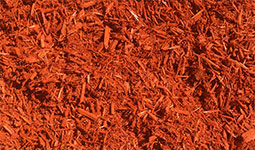# Mulch Order

Get your 2019 mulch order started online with Ack Ack Nursery Company today! We will contact you with the total and for payment. Simply click on the type of mulch you want to select it, fill out the information in the form below, and click the submit button at the bottom. If you have any questions regarding our services, please call us at 815-282-8733.

How much mulch do I need?

Measure each area individually, length x width to calculate your square footage of each area. After the square footage of each area is figured individually, add these results together. Then simply type this square footage total into our mulch calculator below and select your desired depth, click here.
(3" - 4" base recommended)

To manually figure use the following info:

Measure each area individually, length x width to calculate your square footage of each area. After the square footage of each area is figured individually, add these results together, and divide your total by the square footage of the desired depth below. The divided total will be the total number of cubic yards needed.

One cubic yard will cover:
81 square feet @ 4" depth
108 square feet @ 3" depth
162 square feet @ 2" depth
324 square feet @ 1" depth